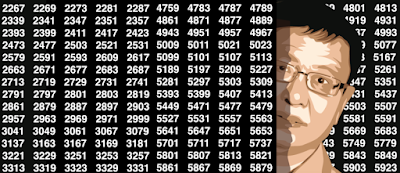## Friday, August 30, 2019

### A Strange Family of Statistical Distributions

I introduce here a family of very peculiar statistical distributions governed by two parameters: p, a real number in [0, 1], and b, an integer > 1.
Potential applications are found in cryptography, Fintech (stock market modeling), Bitcoin, number theory, random number generation, benchmarking statistical tests (see here) and even gaming (see here.) However, the most interesting application is probably to gain insights about how non-normal numbers look like, especially their chaotic nature. It is a fundamental tool to help solve one of the most intriguing mathematical conjectures of all times (yet unsolved): are the digits of standard constants such as Pi or SQRT(2) uniformly distributed or not? For instance, when b = 2, any departure from p = 0.5 (a normal seed) results in a strong discontinuity for f(x) at x = 0.5. If you look at the above chart, f(0) = f(1/2) = f(1) regardless of p, but discontinuities are masking this fact.

### Extreme Events Modeling Using Continued Fractions

Continued fractions are usually considered as a beautiful, curious mathematical topic, but with applications mostly theoretical and limited to math and number theory. Here we show how it can be used in applied business and economics contexts, leveraging the mathematical theory developed for continued fraction, to model and explain natural phenomena.
The interest in this project started when analyzing sequences such as x(n) = { nq } = nq - INT(nq) where n= 1, 2, and so on, and q is an irrational number in [0, 1] called the seed. The brackets denote the fractional part function. The values x(n) are also in [0, 1] and get arbitrarily close to 0 and 1 infinitely often, and indeed arbitrarily close to any number in [0, 1] infinitely often. I became interested to see what happens when it gets very close to 1, and more precisely, about the distribution of the arrival times t(n) of successive records. I was curious to compare these arrival times with those from truly random numbers, or from real-life time series such as temperature, stock market or gaming/sports data. Such arrival times are known to have an infinite expectation under stable conditions, though their medians always exist: after all, any record could be the final one, never to be surpassed again in the future. This always happens at some point with the sequence x(n), if q is a rational number -- thus our focus on irrational seeds: they yield successive records that keep growing over and over, without end, although the gaps between successive records eventually grow very large, in a chaotic, unpredictable way, just like records in traditional time series.
Content:
• Theoretical background (simplified)
• Generalization and potential applications to real life problems
• Original applications in music and probabilistic number theory

### Machine Learning Perspective on the Twin Prime Conjecture

This article focuses on the machine learning aspects of the problem, and the use of pattern recognition techniques leading to interesting,...